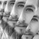TradingView7195 views
This study is an experiment designed to identify market phases using changes in an approximate Hurst Exponent .
The exponent in this script is approximated using a simplified Rescaled Range method.
First, deviations are calculated for the specified period, then the specified period divided by 2, 4, 8, and 16.
Next, sums are taken of the deviations of each period, and the difference between the maximum and minimum sum gives the widest spread.
The rescaled range is calculated by dividing the widest spread by the standard deviation of price over the specified period.
The Hurst Exponent is then approximated by dividing log(rescaled range) by log(n).

The theory is that a system is persistent when the Hurst Exponent value is above 0.5, and antipersistent when the value is below 0.5.

The color scheme indicates 4 different phases I found to be significant in this formula:
- Stabilization Phase
- Destabilization Phase
- Chaos Increase Phase
- Chaos Decrease Phase

This script includes two visualization types to choose from:
- Bar Counter Mode, which displays the number of bars the exponent is consecutively in each phase.
- Hurst Approximation Mode, which displays the approximated exponent value.

Custom bar colors are included.

Please note: This is a rough estimate of the Hurst Exponent . It is not the actual exponent . Numerous approximations exist, and their results all differ slightly.
Open-source script

In true TradingView spirit, the author of this script has published it open-source, so traders can understand and verify it. Cheers to the author! You may use it for free, but reuse of this code in a publication is governed by House Rules. You can favorite it to use it on a chart.

Want to use this script on a chart?
For my full list of premium tools, check the blog:
https://wallanalytics.com/

Reach out on Telegram:
https://t.me/DonovanWall

## CommentsThis is high quality work and great value!
Thank you very much for choosing to share.
ReplyLove the work, this is a very interesting study. Much appreciate you making it public. I've found some promising results when combined with hurst channels. Have a question regarding the bar counter visualisation mode and how you relate your signal descriptions with the theory of the hurst exponent.
In the code

//Threshold
th = 0.5

//Signals
stabilizing = (h > th) and (h > h) ? 1 : 0
destabilizing = (h > th) and (h < h) ? 1 : 0
chaos_increase = (h < th) and (h < h) ? 1 : 0
chaos_decrease = (h < th) and (h > h) ? 1 : 0

A value of over 0.5 is meant to be trend reinforcing and under 0.5 to be a more mean reverting/consolidation period. When I use the indicator the destabilising and chaos_increase periods do correlate to more of a trend/breakout trading situation, whereas the stabilising and chaos_decreasing periods are typically more mean reverting. Also the scale is from zero to 100/200 + not from 0-1.I Maybe I'm missing something later in the code that explains this situational difference to the typical behaviour of the market to the hurst exponent threshold value. If you could shed some more light on this more me it would be much appreciated. Cheers
ReplyTJ_667
@TJ_667,
You can see the three market cycles lining up well with the stabilizing(consolidation) and destabilizing (trend).
ReplyTJ_667
@TJ_667, In regards to your question, when you're using the counter mode, there is no bounded scale. It will simply count how long the phase is active. Here's an extremely unlikely example scenario that will provide you with some clarity (hopefully). If the detected phase was the same throughout the entire series, which again would probably never happen, you would end up seeing a counter value of 10k+ (20k if you're premium) since it's counting how long the phase is active.

Whereas with the H approximation, it is indeed bounded from 0 to 1 since it's reflecting the actual approximate value.

The key difference just lies in the visualization approach. I hope that makes sense.

One key thing to note with H in this script is that it's a crude, very light RS approximation. Now that arrays are implemented in Pine, I plan on doing some rework on this to make the approximation more robust.

And something else to keep in mind with this is that, much like fractal dimension, H is a gauge of complexity, and roughly relates to fractal dimension by the relationship H = 2 - D. So when you analyze this, you're looking for how convoluted the data stream is over the course of the sample size. As you can see in your screenshot, price begins acting a bit more "linear" as the stabilization phase is active, thus suggesting potential trendy behavior. And as the destabilization phase is active, you can see that price begins acting more "planar" with more oscillatory behavior.

I hope this helps!
ReplyDonovanWall
@DonovanWall, thanks man. I wasn't making a distinction between the different scales on the two modes. Great explanation, helps a lot. If you ever do finish up some more work on the hurst exponent I'd love to try it.
Reply